# Chapter Two Bits, Bytes, and Binary

A one or a zero might not mean much to you, but to a computer it means quite a bit. Binary numbers, as you may already know, are numbers made up solely of ones and zeros. And they're the only kind of numbers that a computer can understand. A computer, or at any rate, a digital computer, which is what your Atari is, has great difficulty conceiving of concepts in shades of gray. To a computer, a switch is on or it's off. An electrical signal is there or it isn't. Every thing in a microcomputer's small mind is black or white, plus or minus, off or on.

Call it what you like. It doesn't matter. It's male and female, Shiva and Shakti, yin and yang. Mathematicians sometimes call it Boolean algebra. Computer designers sometimes call it two-state logic. And programmers often refer to it as the binary system. In the binary system, the digit 1 symbolizes the positive, a current that's flowing, for instance, or a switch that's on. The digit 0 represents the negative, a current that's not flowing, or a switch that's off. But there is no digit for the number 2. The only way to represent the number 2 in binary is to take a 1, move it on space to the left, and follow it with a 0, like this: 10. That's right. In binary notation, "10" means 2, not 10, "11" means 3, "100" is 4, "101" is 5, and "110" is 6, and so on.

## Penguin Math

If binary numbers baffle you, a course in Penguin Math might help. Imagine you were a penguin, living on an ice flow. Now penguins don't have 10 fingers on each hand, as people do. Instead, they have two flippers. So if you were a penguin, and counted on your flippers like some people count on their fingers, you'd be able to count only to 2. If you were a very bright penguin, however, you might one day figure out how to use your flippers to count past 2. Suppose, for example, that you decided to equate a raised right flipper to 1, and a raised left flipper to 2. Then you could let two raised flippers be equal to 3. Now suppose you were an extraordinarily bright penguin, and devised a notation system to express in writing what you had done. You could use a 0 to represent an unraised flipper, and a 1 to represent a raised one. And then you could scratch these equations in to ice:

## Penguin Numbers

```00=0
01=1
10=2
11=3
```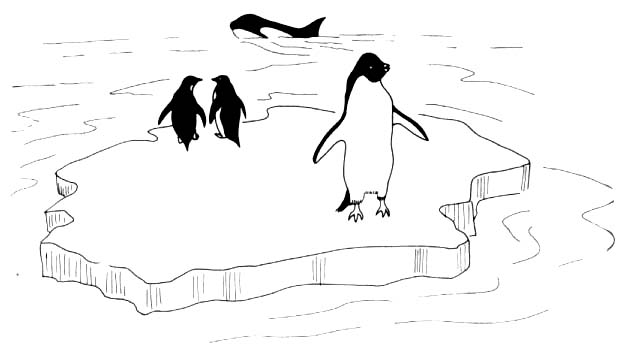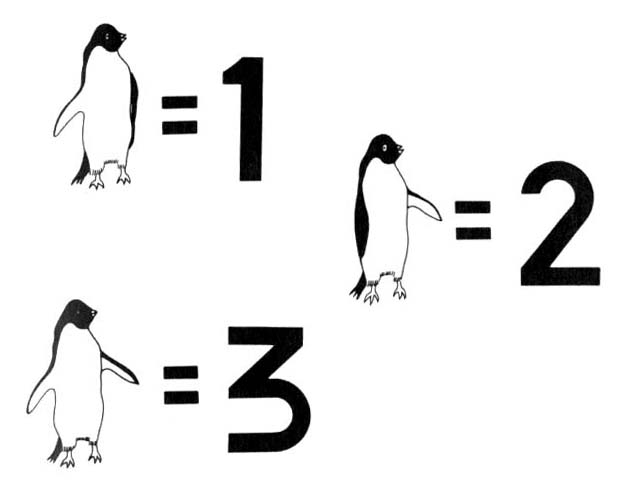Those, of course, are binary numbers. And what they show, in Penguin Math, is that you can express four values - 0 through 3 - as a 2-bit (two-digit) binary number. Now let's suppose that you, as a penguin, wanted to learn to count past 3. Let's imagine that you looked down at your feet and noticed that you had two more flippers. Voila, bigger numbers! If you sat down on your ice flow so that you could raise both arms and both legs at the same time, you could count as follows:

```0000=0
0001=1
0010=2
0011=3
0100=4
0101=5
0110=6
0111=7
1000=8
1001=9
```

... and so on.

If you continue counting like this you would eventually discover that you could express 16 values, 0 through 15, using 4-bit numbers. There's just one more lesson in Penguin Math. Imagine that you, as a penguin, have gotten married to another penguin. And, using your skill with binary numbers, you have determined that you and your spouse have a total of eight flippers between you. If your spouse decides to cooperate with you in counting with flippers, the two of you could now sit on your ice and start a flow chart with numbers that looked like these:

```0000 0001=1
0000 0010=2
0000 0011=3
0000 0100=4
0000 0101=5
```

If you and your spouse kept on counting in this fashion, using 8-bit Penguin Math, you would eventually discover that by using eight flippers you could count from 0 to 255, for a total of 256 values. That completes our brief course in Penguin Math. What it has taught us is that it is possible to express 256 values, from 0 to 255, using 8-bit binary numbers.

## Bits, Bytes and Nibbles

As was pointed out a few paragraphs back, when ones and zeros are used to express binary numbers, they are known as bit. A group of eight bits is called a byte. And a group of four bits is called a nibble (sometimes spelled "nybble"). And a group of 16 bits is called a word. Now we're going to take another look at a series of 8-bit bytes. Observe them closely, and you'll see that every binary number that ends in zero is twice as large as the previous round number; or, in other words, is the square of the previous round number:

```0000 0001 = 1
0000 0010 = 2
0000 0100 = 4
0000 1000 = 8
0001 0000 = 16
0010 0000 = 32
0100 0000 = 64
1000 0000 = 128
```

Here are two more binary numbers that assembly language programmers often find worth memorizing:

```1111 1111 = 255
1111 1111 1111 1111 = 65,535
```

T he number 255 is worthy of note because it's the largest 8-bit number. The number 65,535 is the largest 16-bit number. Now the plot thickens. As we have mentioned, Atari computers are called 8-bit computers because they're built around an 8-bit microprocessor, a computer processor chip that handles binary numbers up to eight places long, but no longer. Because of this limitation, your Atari can't perform calculations on numbers larger than 255, in fact it can't even perform a calculation with a result that's greater than 255!

Obviously, this 8-bit limitation places severe restrictions on the ability of Atari computers to perform calculations on large numbers. In effect, the 6502's Arithmetic Logic Unit (ALU) is like a calculator that can't handle a number larger than 255. There are ways to get around that limitation, of course, but it isn't easy. To work with numbers larger than 255, an 8-bit computer has to perform a rather complex series of operations. If a number is greater than 255, an 8-bit computer has to break it down into 8-bit chunks, and perform each required calculation on each 8-bit number. Then the computer has to patch all of these 8-bit numbers back together again.

If the result of a calculation is more than eight bits long, things get even more complicated. That's because each memory location in an 8-bit computer, each cell in its Random Access Memory (RAM) as well as its Read Only Memory (ROM), is an 8-bit memory register. So if you want to store a number larger than 255 in an 8-bit computer's memory, you have to break it up into two or more 8-bit numbers, and then store each of those number in a separate memory location. And then, if you ever want to use the original number again, you have to patch it back together from the 8-bit pieces it was split into.

## 8-bit Versus 16-bit Computers

Now that you know all that, you can understand why 16-bit computers, such as the IBM Personal Computer and its many imitators, can run faster than 8-bit computers can. A 16-bit computer can handle binary numbers up to 16 bits long without doing any mathematical cutting and pasting, and can there fore process numbers ranging up to 65,535 in single chunks, a 16-bit word at a time. So 16-bit computers are considerably faster than 8-bit computers are, at least when they're dealing with large numbers. 16-bit computers also have another advantage over 8-bit computers. They can keep track of much more data at one time than 8-bit computers can. And they can therefore be equipped with much larger memories.

A computer's memory can be visualized as a large grid containing thousands of pigeonholes, or memory locations. In an Atari computer, each of those locations can hold one 8-bit number. Earlier, we spoke of an analogy between a computer's memory and tiers upon tiers of post office boxes. Now we can extend that analogy a little further.

But there's still a limit to the number of address locations that an 8-bit computer can keep track of. Since 255 is the largest number that an 8-bit register can hold, the X axis of an address can range only from 0 to 255, an total of 256 numbers. The same limit applies to the Y axis. So unless certain fancy memory expansion tricks are used, the maximum memory capacity of an 8-bit computer is 256 times 256, or 65,536; in other words, 64K. The reason for the odd number, incidentally, is that 64K equates to a binary number, not a decimal number. 64K is not equal to decimal 64 times decimal 1,000, but is equivalent instead to the product of 64 and 1024. Those two numbers look like they were pulled out of a hat when they're written in decimal notation, but in binary they're both nice round numbers: 0100 000 and 0100 000 000, respectively.

## What a Difference 8 Bits Make

If a 16-bit computer kept track of the addresses in its memory the same way an 8-bit computer does, by using the X axis and the Y axis of each location as reference points, then a 16-bit computer could address more than 4 million memory locations (65,536 cells y 65,536 cells). But 16-bit computers don't usually keep track of the address in their memories that way. In the IBM-PC for example, each memory location is assigned what amounts to a 20-bit address. So an IBM-PC can address 1,048,576 address locations; not quite the 4 million plus locations that it could address with an X,Y matrix system, but still enough locations to hold more than a million bytes (a megabyte) of memory.

Now you can understand what all of the fuss over 16-bit computers is all about. 16-bit computers can address more memory than 8-bit computers can, and can also process information faster. In addition, they're easier to program in assembly language than 8-bit computers are, since they can digest chunks of data that are 16 bits long. Since Atari are 8-bit computers, none of this talk about 16-bit computers is likely to help you much in your quest for knowledge about Atari assembly language. Fortunately, however, a knowledge of Atari assembly language will help you a great deal if you ever decide to study a 16-bit assembly language. If you can learn to juggle bits well enough to become a good Atari assembly language programmer, you'll probably find that 16-bit assembly language, which doesn't require any splicing together of 8-bit numbers, is a snap to learn.

What's the sum of D plus F? Well, it's 1C if you're working in hexadecimal numbers.

Hexadecimal numbers as you may know if you've done much programming, are strange looking combinations of letters and numbers that are often used in assembly language programs. The hexadecimal notation system uses not only the digits 0 through 9, but also the letters A through F. So weird looking letter and number combinations like FC3B, 4A5D and even ABCD are perfectly good numbers in the hexadecimal system. Hex numbers are often used by assembly language programmers because they're closely related to binary numbers. It's that close relationship that we're going to take a look at now.

## 16-Finger Math

Remember how we used Penguin Math to explain the concept of binary numbers? Well, if you can imagine that you lived in a society where everyone had 16 fingers instead of 10 fingers like us, or two flippers like a penguin, then you'll be able to grasp the concept of hexadecimal numbers quite easily. Binary numbers as we have pointed out, have a base of 2. Decimal numbers, the kind we're used to, have a base of 10. And hexadecimal numbers have a base of 16. Hexadecimal numbers are used in assembly language programming because they're quite similar to binary numbers; which, as we pointed out in Chapter 1, are the kind of numbers that computers understand. At first glance it may be difficult to see how binary numbers and hexadecimal numbers have anything in common. But you can see very clearly how binary and hex numbers relate to each other simply by looking at this chart:

```Decimal      Hexadecimal    Binary

0               0        0000 0000
1               1        0000 0001
2               2        0000 0010
3               3        0000 0011
4               4        0000 0100
5               5        0000 0101
6               6        0000 0110
7               7        0000 0111
8               8        0000 1000
9               9        0000 1001
10              A        0000 1010
11              B        0000 1011
12              C        0000 1100
13              D        0000 1101
14              E        0000 1110
15              F        0000 1111
16             10        0001 0000
```

As you can see from this list, the decimal number 16 is written "10" in hex and "0001 0000" in binary, and is thus a round number in both systems. And the hexadecimal digit F, which comes just before hex 10 (or 16 in decimal), is written 00001111 in binary. As you become more familiar with the binary and hexadecimal systems, you will begin to notice many other similarities between them. For example, the decimal number 255 (the largest 8-bit number) is 1111 1111 in binary and FF in hex. The decimal number 65,535 (the highest memory addresses in a 64K computer) is written FFFF in hex and 1111 1111 1111 1111 in binary. And so on. The point of all this is that it's much easier to convert back and forth between binary and hexadecimal numbers than it is to convert back and forth between binary and decimal numbers.

```1011 1000 Binary
184       Decimal

0010 1110 Binary
46        Decimal

1111 1100 Binary
252       Decimal

0001 1100 Binary
28        Decimal
```

As you can see, a nibble (four bits) in binary notation always equates to one digit in hexadecimal notation. But there is no clear relationship between the length of a binary number and the length of the same number written in decimal notation. This same principle can be extended to binary, hexadecimal and decimal conversions of 16-bit numbers. For example:

```1111 1100 0001 1100 Binary
64540               Decimal
```

## Some Illustrative Programs

Now we’ll look at some BASIC programs that perform operations involving binary, decimal and hexadecimal numbers. Since all Atari computers are 8-bit computers (at this writing, anyway), the only way to store a 16-bit number in an Atari is to put it into two memory registers. And 6502-based computers use the odd (to some people) convention of storing 16-bit numbers with the lower (least significant) byte first and the higher (most significant) byte second. For example, if the hexadecimal number FC1C were stored in hexadecimal memory address 0600 and 0601, FC (most significant byte) would be stored in address 0601, and 1C (the least significant byte) would be stored in address 0600. (In assembly language programs, incidentally, hexadecimal numbers are usually preceded with dollars signs so that they can be distinguished from decimal numbers. The hexadecimal addresses 0600 and 0601, therefore, would ordinarily be written \$0600 and \$0601 in an assembly language program.

Now let’s suppose, just for illustration purposes, that you wanted to store a 16-bit number in two 8-byte address in your computer’s RAM (\$0600 and \$0601 for example), while running a BASIC program. Since BASIC programs are written using ordinary decimal numbers, the first thing you’d have to do is convert the addresses you were going to be working with, \$0600 and \$0601, into decimal numbers. You could do that in a number of different ways. You could look up the decimal equivalents of \$0600 and \$0601 on a decimal/hexadecimal conversion chart. Or you could carry out the necessary conversions by hand. Or you could perform them with the help of a computer program. No matter how you managed to make the conversions, however, what you would wind up discovering is that the hexadecimal number \$0601 is equivalent to decimal 1537. Once you found this out, you could store the 16-bit value in \$0600 and \$0601 using the following BASIC routine (or some variation of the same theme):

### Routine for Storing a 16-bit Number in RAM STORE16.BAS

```10 PRINT "TYPE A POSITIVE  INTEGER"
20 PRINT "RANGING FROM 0 TO 65,535"
30 INPUT X
40 LET HIBYTE=INT(X/256)
50 LET LOBYTE=X-HIBYTE*256
60 POKE 1536,LOBYTE
70 POKE 1537,HIBYTE
80 END
```

####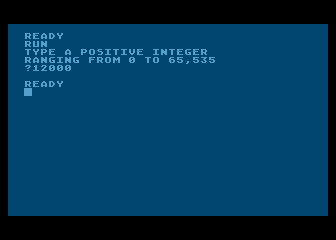Screen Shot Of STORE16.BAS

Now let’s assume that you wanted to retrieve a 16-bit number stored in your computer’s RAM; for example, the number stored in memory addresses \$0600 and \$0601 in the above program. And let’s suppose, once again, that you wanted to do that while you were running a BASIC program. Your BASIC routine might look something like this:

### Retrieving a 16-Bit Number from RAM RETR16.BAS

```10 LET X=PEEK(1537)*256+PEEK(1536)
20 PRINT X
```

## Converting Binary Numbers to Decimal Numbers

Since assembly language programmers work with three different kinds of numbers, decimal, hexadecimal and binary numbers, they often find it necessary to perform conversions between one number base and another. It isn’t very difficult to convert a binary number to a decimal number. In a binary number, the bit farthest to the right represents 2 to the power 0. The next bit to the left represents 2 to the power 1, the next represents 2 to the power 2, and so on. The digits in an 8-bit binary number are therefore numbered 0 to 7, starting from the rightmost digit. The rightmost bit – often referred to as the least significant bit, or LSB - represents 2 to the 0th power, or the number 1. And the leftmost bit - often called the most significant bit, or MSB - is equal to 2 to the 7th power, or 128. Here’s a list of simple equations that illustrate what each bit in an 8-bit binary number means:

```Bit 0 = 2^0 = 1
Bit 1 = 2^1 = 2
Bit 2 = 2^2 = 4
Bit 3 = 2^3 = 8
Bit 4 = 2^4 = 16
Bit 5 = 2^5 = 32
Bit 6 = 2^6 = 64
Bit 7 = 2^7 = 128
```

Using the above chart, it’s easy to convert any 8-bit binary number into its decimal equivalent. Instead of writing the number down from left to right, write it instead in a vertical column, with bit 0 at the top of the column and bit 7 at the bottom. Then multiply each bit in the binary number by the decimal number that it represents. Then add up the results of all of these multiplications. The total you get will be the decimal value of the binary number. Suppose, for example, that you wanted to convert the binary number 00101001 into a decimal number. Here’s how you’d do it:

```1 X   1 =  1
0 X   2 =  0
0 X   4 =  0
1 X   8 =  8
0 X  16 =  0
1 X  32 = 32
0 X  64 =  0
0 X 128 =  0
------------
TOTAL   = 41
```

According to the results of this calculation, the binary number 00101001 is equivalent to the decimal number 41. Look up either 00101001 or 41 on a binary-to=decimal or decimal-to-binary conversion chart, and you’ll see that the calculation was accurate. And this conversation technique will work with any other binary number. Now we’ll go in the other direction, and convert a decimal number to a binary number. And here’s how we’ll do that: We’ll divide the number by 2, and write down both the quotient and the remainder. Since we’ll be dividing by 2, the quotient will be either a 1 or 0. So we’ll write down either a 1 or a 0. Then we’ll take the quotient we got, divide it by two, and write that quotient down. If there’s a remainder (a 1 or a 0), we’ll write that down, too, right underneath the first remainder. When there are no more numbers left to divide, we’ll write down all of the remainders we got, reading from the bottom to the top. What we’ll have then, of course, is a binary number, a number made up of ones and zeros. And that number will be the binary equivalent of the decimal number we started out with. Now let’s try this conversion technique on the decimal number 117:

```117/2 = 58 with a remainder of 1
58/2 = 29 with a remainder of 0
29/2 = 14 with a remainder of 1
14/2 =  7 with a remainder of 0
7/2 =  3 with a remainder of 1
3/2 =  1 with a remainder of 1
1/2 =  0 with a remainder of 1
```

According to the results of this calculation, the binary equivalent of the decimal number 117 is 01110101. And this result, as a check of a decimal-to-binary conversion chart would confirm, is also accurate.

## Binary-to-Hex and Hex-to-Binary Conversions

It’s easy to convert binary numbers to their decimal equivalents. Just use this chart:

```HEXADECIMAL	BINARY

0		0000
1		0001
2		0010
3		0011
4		0100
5		0101
6		0110
7		0111
8		1000
9		1001
A		1010
B		1011
C		1100
D		1101
E		1110
F		1111
```

To convert a multiple digit hex number to binary, just string the hex digits together and convert each one separately. For example, the binary equivalent of the hexadecimal number C0 is 1100 0000. The binary equivalent of the hex number 8F2 is 1000 1111 0010. The binary equivalent of the hex number 7A1B is 0111 1010 0001 1011. And so on. To convert binary numbers to hexadecimal numbers, just use the chart in reverse. The binary number 1101 0110 1110 0101, for example, is equivalent to the hexadecimal number D6E5.

## Doing It the Easy Way

Even though it isn’t difficult to convert binary numbers to hexadecimal and vice versa, it is time consuming to do it by hand, and when you program in assembly language, you have to do a lot of binary-to-decimal and decimal-to-binary converting. So there are a lot of BASIC programs around for converting numbers back and forth between the binary and decimal notation systems. And now I’m going to give two of them to you, absolutely free. Here’s one for converting binary numbers to decimal numbers:

### Converting Binary Numbers To Decimal Numbers BIN2DEC.BAS

```5 REM ** BINARY-DECIMAL CONVERSION **
7 REM ** BIN2DEC.BAS **
10 DIM BN\$(9),BIT(8),T\$(1)
20 GRAPHICS 0
25 ? :? "BINARY-DECIMAL CONVERSION"
30 ? :? "ENTER AN 8-BIT BINARY NUMBER:":? :INPUT BN\$
35 IF LEN(BN\$)<>8 THEN 30
40 FOR L=1 TO 8
50 T\$=BN\$(L)
55 IF T\$<>"0" AND T\$<>"1" THEN 30
60 BIT(L)=VAL(T\$)
70 NEXT L
75 ANS=0
80 M=256
90 FOR X=1 TO 8
100 M=M/2:ANS=ANS+BIT(X)*M
110 NEXT X
140 ? "DECIMAL: ";ANS
150 GOTO 30
```

####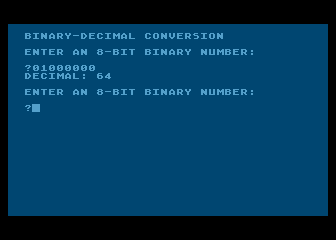Screen Shot Of BIN2DEC.BAS

And here’s a program for converting decimal numbers to binary numbers:

### Converting Decimal Numbers To Binary Numbers DEC2BIN.BAS

```5 REM ** DECIMAL-BINARY CONVERSION **
7 REM ** DEC2BIN.BAS **
10 DIM BIN\$(8),TEMP\$(8),R\$(1)
20 GRAPHICS 0
30 ? :? "DECIMAL-BINARY CONVERSION"
40 ? :? "ENTER A POSITIVE INTEGER (0 TO 255):":? :TRAP 40:INPUT NR
50 IF NR-INT(NR)<>0 THEN 40
60 IF NR>255 OR NR<0 THEN 40
70 FOR L=1 TO 8
80 Q=NR/2
90 R=Q-INT(Q)
100 IF R=0 THEN R\$="0":GOTO 120
110 R\$="1"
120 TEMP\$(1)=R\$:TEMP\$(2)=BIN\$:BIN\$=TEMP\$
130 NR=INT(Q)
140 NEXT L
150 ? "BINARY: ";BIN\$
160 TRAP 40000
170 GOTO 40
```

####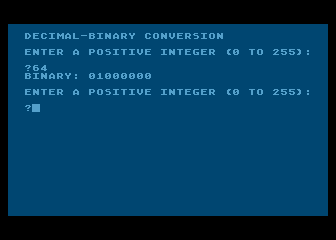Screen Shot Of DEC2BIN.BAS

Decimal/hexadecimal conversion is a complex process best done on a computer or a special calculator. Texas Instruments makes a calculator called the Programmer that can perform decimal hexadecimal conversions in a flash, and can also add, subtract, multiply and divide both decimal and hexadecimal numbers. Many assembly language program designers use the TI Programmer, or some similar calculator, and would have a hard time getting along without it. In case you can’t get your hands on a programmer’s calculator right away, here’s an Atari BASIC program that will convert decimal numbers to hexadecimal numbers and vice versa. Another program is available on page H-18 of the BASIC Reference Manual that comes with the Atari BASIC cartridge.

### Dec-Hex and Hex-Dec Conversion Program HEX2DEC.BAS

```10 REM
20 REM HEX TO DEC CONVERSION PROGRAM
30 REM
40 REM THE FAST WAY
50 REM DON'T USE MATH
60 REM
70 DIM H\$(40),A\$(40)
80 REM
90 PRINT
100 PRINT
110 PRINT
120 PRINT "HEX TO DECIMAL CONVERSION"
130 PRINT
140 PRINT "H) HEX TO DEC"
150 PRINT "D) DEC TO HEX"
160 PRINT
180 INPUT A\$
190 IF A\$="H" THEN 220
200 IF A\$="D" THEN 400
210 GOTO 100
220 REM
230 REM CONVERT HEX TO DECIMAL
240 REM
250 PRINT "ENTER HEX NUMBER";
260 INPUT H\$
270 REM
280 D=0
290 S=1:REM POSITIONAL MULTIPLIER
295 REM GO THROUGH THE STRING
300 FOR L=LEN(H\$) TO 1 STEP -1
310 A\$=H\$(L,L)
320 REM
330 REM CONVERT "0"-"F" TO 0-15
340 N=ASC(A\$)-48:IF N>9 THEN N=N-7
345 IF N<0 OR N>15 THEN 90
350 D=D+N*S
360 S=S*16
370 NEXT L
380 PRINT "HEX ";H\$;"= DEC ";D
390 GOTO 90
400 REM
410 REM CONVERT DECIMAL TO HEX
420 REM
430 PRINT "ENTER DECIMAL TO CONVERT"
440 INPUT D
450 REM
460 REM FIRST FIND THE HIGHEST DIGIT
470 REM
480 S=16
490 X=2
500 IF S<D THEN X=X+1:S=S*16:GOTO 500
505 PRINT "DECIMAL ";D;" = HEX ";
510 T=D
520 FOR L=X TO 1 STEP -1
530 N=INT(T/S)
540 PRINT CHR\$(48+N+7*(N>9));:REM CONVERT DEC TO HEX 0-F
550 T=T-N*S:S=S/16
560 NEXT L
570 PRINT
580 GOTO 90
```

####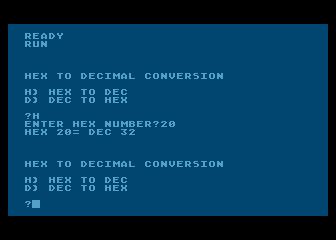Screen Shot Of HEX2DEC.BAS

That concludes our crash course into bits, bytes, and binary. This was an important chapter because it’s a prerequisite to Chapter 3, which in turn is a prerequisite to Chapter 4, where you’ll get a chance to actually start writing some assembly language programs.

## Something to Tide You Over

Meanwhile, here’s another BASIC program that might help you feel that you’re getting through to your Atari. It uses an infinite loop to cycle through all of the colors and hues that your computer can generate, loading each of them in turn into the memory register that controls the boarder area of your video screen. In Chapter 9, Programming Bit by Bit, you’ll learn how to do this same trick using assembly language.

### Bonus Program No. 2 The Atari Rainbow RAINBOW.BAS

```10 REM ** THE ATARI RAINBOW **
20 REM ** RAINBOW.BAS" **
30 REM
40 FOR L=2 TO 254 STEP 2:REM ALL VALID COLORS HAVE EVEN NUMBERS
50 POKE 712,L:REM 712 IS ADDRESS OF BORDER AREA COLOR REGISTER
60 FOR WAIT=1 TO 10:NEXT WAIT:REM JUST A DELAY LOOP TO USE UP TIME
70 NEXT L
80 GOTO 40:REM AN INFINITE LOOP
```

####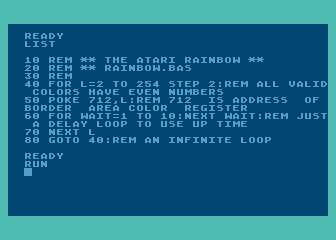Screen Shot Of RAINBOW.BAS

Type this program into your computer, run it, and watch the show! Then we’ll continue on to Chapter 3.